Search test library by skills or roles
⌘ K

# Adaface Sample Technical Aptitude Questions

Here are some sample Technical Aptitude questions from our premium questions library (10273 non-googleable questions).

### Skills

#### Accounting

🧐 Question

Medium

Decryptor
Strings
Solve
```                                    What does the following pseudo code print?
```

Options:

• cfw k
• abcde
• abc e
• aaaaaa
• aaa a
• cfwk
• cfxl
• c f x . l

Medium

Fibonacci codes
Time Complexity
Pseudo Code
Solve
```                                    Here are three pseudocodes for calculating the Nth Fibonacci number. Which of the following statements about these codes are true?
```
```                                    A: Time complexity/efficiency of CODE 1, CODE 2, CODE 3 is the same.
B: CODE 2 is more space-efficient than CODE 3.
C: CODE 3 is more space-efficient than CODE 2.
D: CODE 2 is more time-efficient than CODE 1.
E: CODE 3 is more time-efficient than CODE 1.
```

Options:

• B D
• B E
• C D
• C E
• A B D
• A B E
• A C D
• A C E
• B D E
• C D E
• A B D E
• A C D E
• A B C D E

Medium

Palindrome Test cases
Strings
Solve
```                                    Go through the following faulty palindrome detection pseudocode and test cases. For which of the test cases would the code return true?
```
```                                    Testcases
A: a
B: aba
C: aaa
D: aac
```

Options:

• A
• B
• C
• D
• A B
• A C
• A D
• B C
• B D
• C D
• A B C
• B C D
• A C D
• A B D
• A B C D

Medium

Rewards Activities Order
Database Connection
Solve
```                                    A new popular app tracks the activities performed by its users and grants them rewards based on each individual's total activities performed in a year. This data is maintained in a database table (activitesinfo), with the following columns: userid, totalactivities, reward. This year, due to fundraising activity, the company has decided to further reward its users by enhancing the reward as per the following formula: If reward < = 4000, increase it by 2%, If 4000 < reward <= 8000, increase it by 4%, If reward > 8000, increase it by 6%.
The tech team has written three different scripts to calculate increment for each slab, each script is to run as a separate transaction. Here are the three scripts:
```
```                                    Script 1:
For users with reward <= 4000, set reward = reward * 1.02

Script 2:
For users with reward > 4000 and reward <= 8000, set reward = reward * 1.04

Script 3:
For users with reward > 8000, set reward = reward * 1.06
```
```                                    Each script is to be executed separately. Which of the following options will update the rewards as expected:
```
```                                    A: Execute Script 1 followed by Script 2 followed by Script 3
B: Execute Script 2 followed by Script 3; Script 1 running concurrently throughout
C: Execute Script 3 followed by Script 2; Script 1 running concurrently throughout
D: Execute Script 3 followed by Script 2 followed by Script 1
E: Execute Script 2 followed by Script 3 followed by Script 1
```

Options:

• A
• B
• C
• D
• E
🧐 Question🔧 Skill

Medium

Decryptor
Strings
2 mins
Technical Aptitude
Solve

Medium

Fibonacci codes
Time Complexity
Pseudo Code
2 mins
Technical Aptitude
Solve

Medium

Palindrome Test cases
Strings
2 mins
Technical Aptitude
Solve

Medium

Rewards Activities Order
Database Connection
3 mins
Technical Aptitude
Solve
🧐 Question🔧 Skill💪 Difficulty⌛ Time
Decryptor
Strings
Technical Aptitude
Medium2 mins
Solve
Fibonacci codes
Time Complexity
Pseudo Code
Technical Aptitude
Medium2 mins
Solve
Palindrome Test cases
Strings
Technical Aptitude
Medium2 mins
Solve
Rewards Activities Order
Database Connection
Technical Aptitude
Medium3 mins
Solve

## Adaface questions are trusted by enterprises globally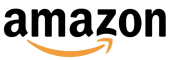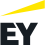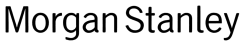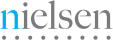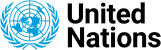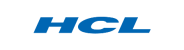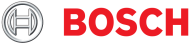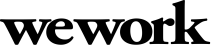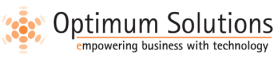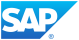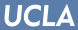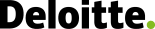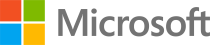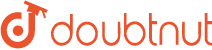We evaluated several of their competitors and found Adaface to be the most compelling. Great default library of questions that are designed to test for fit rather than memorization of algorithms.

Swayam Narain, CTO, AffableJoin 1200+ companies in 75+ countries.